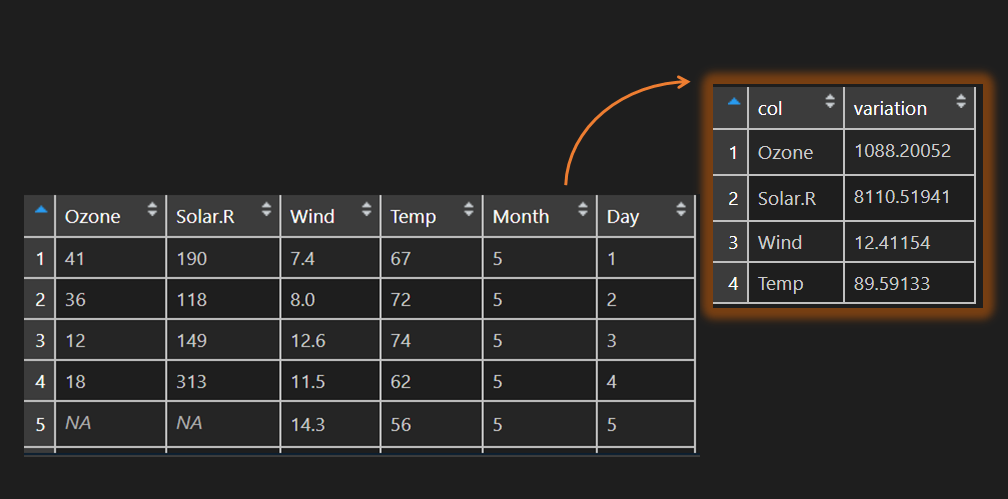# Variance or other calculation across columns in R

Here is how to calculate variance across columns in the R data frame. The same technique can be useful in other situations. Like in this other example. Get a count of NA values for each of the columns in the R data frame.

A variance of the features might be important in machine learning and can help you better select necessary ones. Sometimes some of them that have variance measurement near to zero might be unnecessary.

Here are the first rows of the airquality dataset. There are missing values that should be taken into consideration. Luckily in variance calculation is a na.rm argument to deal with that. All it takes is to know how to use it in apply family functions.

```head(airquality)
#  Ozone Solar.R Wind Temp Month Day
#1    41     190  7.4   67     5   1
#2    36     118  8.0   72     5   2
#3    12     149 12.6   74     5   3
#4    18     313 11.5   62     5   4
#5    NA      NA 14.3   56     5   5
#6    28      NA 14.9   66     5   6```

Here is the variance calculation across the first four columns of the R airquality dataset by using lapply. Cbind will combine them together.

```cbind(
lapply(airquality[1:4], FUN = var, na.rm = T)
)

#        [,1]
#Ozone   1088.201
#Solar.R 8110.519
#Wind    12.41154
#Temp    89.59133```

If you would like to get R data frame column variance in one simple data frame, you can do that like this.

```x <- cbind(lapply(airquality[1:4], FUN = var, na.rm = T))

vardf <- data.frame('col' = rownames(x), 'variation' = unlist(x))

vardf
#      col  variation
#1   Ozone 1088.20052
#2 Solar.R 8110.51941
#3    Wind   12.41154
#4    Temp   89.59133```

Here is another example with apply function to execute calculations in each row of the data frame.

Take a look at my favorite RStudio tips and tricks. For example, how to fold a part of the R code and use section naming.

Categories R